## Posts

Showing posts from March 13, 2022

### The Diffraction Grating for A Level Physics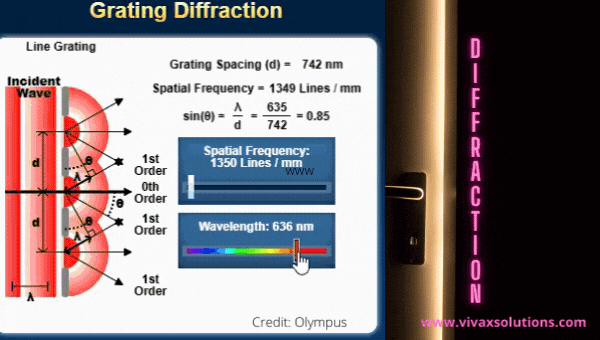Diffraction grating is a glass slide with a series of parallel transparent lines, called slits, on it. When a beam of monochromatic beam strikes it, light waves diffract through the slits, creating a pattern of alternating bright and dark spots, known as fringes, on a surface where light falls - in addition to a central, wider bright spot. At the centre, the light waves meet up in phase. On either side of the central fringe, however, they meet up with with increasing path differences between the waves in question.     For bright fringes, the path differences are multiples of  λ and for the dark fringes that alternate between the bright fringes, the path differences are multiples of  λ/2.

### Fourth Circle Theorem: Cycling Quadrilaterals - GCSE, IGCSE, GCE OL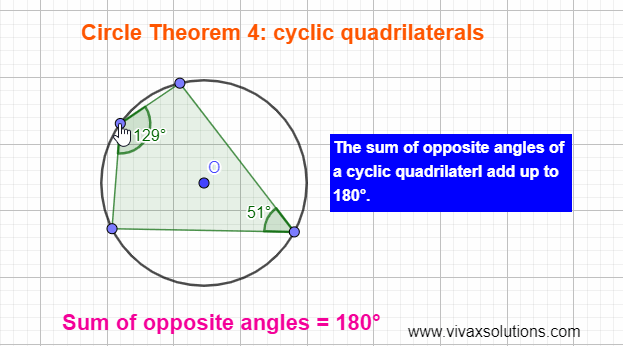Cyclic Quadrilaterals   A quadrilateral whose vertices touch a circle is called a cyclic quadrilateral.  Fourth Circle Theorem The opposite angles of a cyclic quadrilateral add up to 1800. You can practise it interactively with the following applet:

### First three circle theorems for GCSE, IGCSE and GCE OL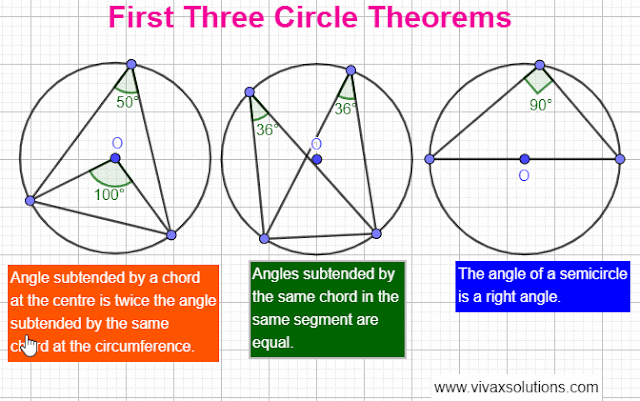The above simulations show the first three circle theorems.     You can practise them interactively with the following simulation:   If you want more interactive circle theorem simulations and worked examples, please click here .

### The net of a cube: for Year 9, GCSE & IGCSE maths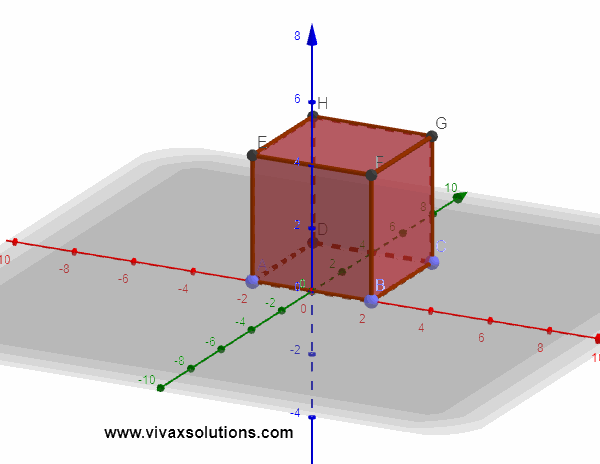The above simulation shows how a cube is formed from a net or vice a versa. The net of a triangular-based prism is as follows: In the GCSE or IGCSE exams, students are often asked to draw a net of a regular solid object such as a prism, cube or even a tetrahedron for which the former are required to have some early exposure to the constructions.

### Maximizing the power of load in an electric circuit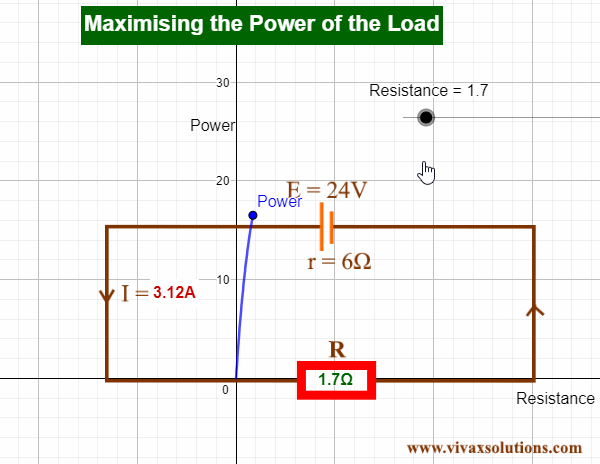Let the EMF, internal resistance, load and the current of a circuit be E, r, R and I respectively. E = I(R + r) I = E/(R + r) The power of the load, the external resistor in this case, is as follows: P = VI P = E 2 R/(R + r) 2 Let's differentiate P with respect to R dP/dR = E 2/ / (R + r) 2 - 2E 2 R / (R + r) 3 When P is maximum,  dP/dR = 0 E 2/ / (R + r) 2 - 2E 2 R / (R + r) 3 = 0 R + r = 2R R = r As you can see, the power is maximum, when the external resistance is equal to the internal resistance of the battery or cell.     E.g.  R = r E = 12V R = 6 r = 6 Power = (12/12) 2 X 6 = 6W R > r E = 12 R  = 12 r = 6 Power = (12/18) 2 X 12 = 5.3W R < r E = 12 R = 4 r = 6 Power = (12/10) 2 X 4 = 5.7 W You can practise the above with the following interactive simulation:     For a comprehensive tutorial on electricity, for GCSE, IGCSE, IB, A Level,  GCE OL and GCE AL, please use this link .

### Conservation of Mechanical Energy: potential and kinetic energy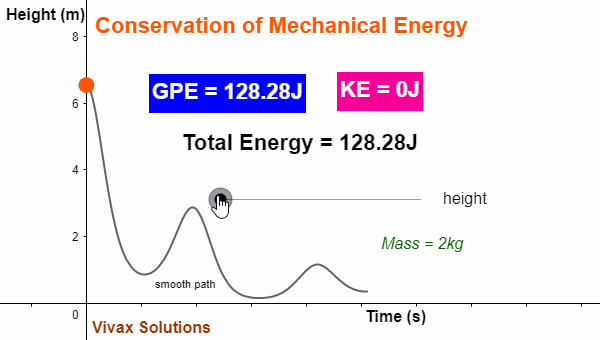Conservation of Mechanical Energy The sum of the kinetic energy and potential energy of an object that moves under gravity remains constant throughout its journey. Kinetic Energy(KE) The energy possessed by a moving object is called Kinetic energy.     If the mass and the velocity of an object are m and v respectively, KE = 1/2 mv 2 Gravitational Potential Energy(GPE) The energy possessed by an object due to its position is called its potential energy. GPE = mgh m, g and h are mass, acceleration due to gravity and height respectively. If an object moves under gravity in the absence of air resistance, according to the Conservation of Mechanical Energy, KE + GPE = constant. You can practise it and see for yourself with the following applet:

### Single Slit Diffraction for A Level Physics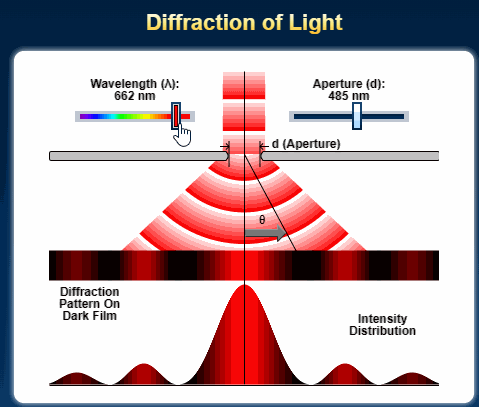Credit: Olympus The diffraction of a beam of light through a single slit is given by the following equation. x = d sin θ, where x is the path difference and θ is the deviation of the path as an angle. d is the width of the slit or aperture.  Single slit diffraction The greater the angle of diffraction, the greater the value of x. If x is a multiple of λ, the wavelength, bright spots are visible on the screen. If x is a multiple of 1/2 λ, however, the spots become dark; at those points, constructive and destructive interference take place respectively.      The spot spread on the screen with an equal gap between them. The values of x for the bright spots can be λ, 2λ, 3λ and so on. For the dark spots, the are 1/2 λ, 3/2 λ, 5/2 λ and so on.  The intensity of the spots, however, goes down with the distance from the central spot, which is twice as wide as other spots. From the above equation,  sin θ = λ/d Based on the above formula, the greater the width of aperture, the small

### Modelling with Parametric Equations: A Level Pure Mathematics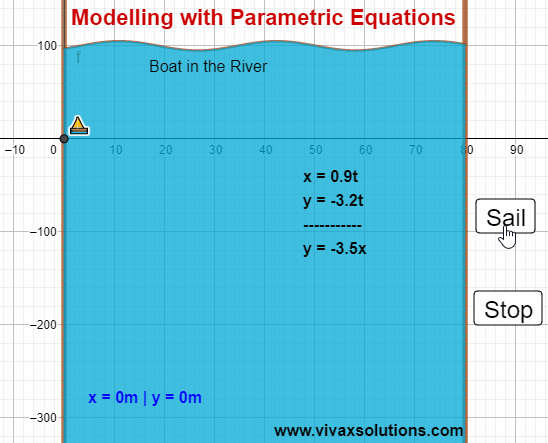Modelling with Parametric Equations for A Level pure mathematics Parametric equations can be used to model real life situations. For example, the above animation shows how the path of a boat in river can be modelled by parametric equations. The boat sails from west to east and the river flows from north to south in this case.  x and y are distances measured from the west and north respectively. If the parameter is time, t, x = 0.9t | y = -3.2t The cartesian equation of the path is derived as follows: x = 0.9t => t = x/0.9 y = -3.2(x/0.9) y = -3.5x Therefore, the path of the boat is a straight line. You can practise the above interactively here: